# CBSE Solutions for Class 10 English

#### GSEB std 10 science solution for Gujarati check Subject Chapters Wise::

The decimal expansion of the rational number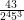will terminate after how many places of decimals? (2013)

જવાબ :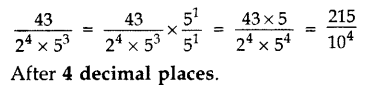Write the decimal form of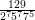જવાબ : Non-terminating non-repeating.

Find the largest number that will divide 398, 436 and 542 leaving remainders 7, 11, and 15 respectively.

જવાબ : Algorithm
398 – 7 = 391, 436 – 11 = 425, 542 – 15 = 527
HCF of 391, 425, 527 = 17

Express 98 as a product of its primes.

જવાબ : 2 × 72

If the HCF of 408 and 1032 is expressible in the form 1032 × 2 + 408 × p, then find the value of p.

જવાબ : HCF of 408 and 1032 is 24.
1032 × 2 + 408 × (p) = 24
408p = 24 – 2064
p = -5

HCF and LCM of two numbers is 9 and 459 respectively. If one of the numbers is 27, find the other number. (2012)

જવાબ : We know,
1st number × 2nd number = HCF × LCM
⇒ 27 × 2nd number = 9 × 459
⇒ 2nd number =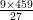= 153

Find HCF and LCM of 13 and 17 by prime factorisation method. (2013)

જવાબ : 13 = 1 × 13; 17 = 1 × 17
HCF = 1 and LCM = 13 × 17 = 221

Find LCM of numbers whose prime factorisation are expressible as 3 × 5and 3× 7(2014)

જવાબ : LCM (3 × 52, 32 × 72) = 32 × 52 × 72 = 9 × 25 × 49 = 11025

Find the largest number which divides 70 and 125 leaving remainder 5 and 8 respectively. (2015)

જવાબ : It is given that on dividing 70 by the required number, there is a remainder 5.
This means that 70 – 5 = 65 is exactly divisible by the required number.
Similarly, 125 – 8 = 117 is also exactly divisible by the required number.
65 = 5 × 13
117 = 32 × 13
HCF = 13
Required number = 13

Find the LCM of 96 and 360 by using fundamental theorem of arithmetic. (2012)

જવાબ : 96 = 25 × 3
360 = 23 × 32 × 5
LCM = 25 × 32 × 5 = 32 × 9 × 5 = 1440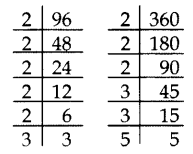Find the HCF (865, 255) using Euclid’s division lemma. (2013)

જવાબ : 865 > 255
865 = 255 × 3 + 100
255 = 100 × 2 + 55
100 = 55 × 1 + 45
55 = 45 × 1 + 10
45 = 10 × 4 + 5
10 = 5 × 2 + 0
The remainder is 0.
HCF = 5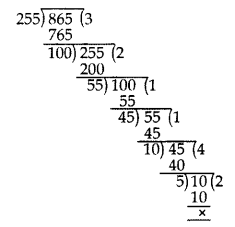Find the prime factorisation of the denominator of rational number expressed as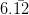in simplest form. (2014)

જવાબ : Let x =…(i)
100x = 612.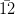…(ii)
…[Multiplying both sides by 100]
Subtracting (i) from (ii),
99x = 606
x =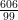=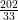Denominator = 33
Prime factorisation = 3 × 11

Complete the following factor tree and find the composite number x. (2014)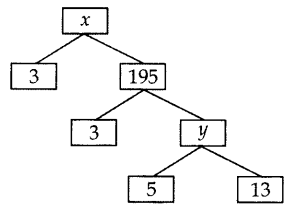જવાબ : y = 5 × 13 = 65
x = 3 × 195 = 585

Prove that 2 + 3√5 is an irrational number. (2014)

જવાબ : Let us assume, to the contrary, that 2 + 3√5 is rational.
So that we can find integers a and b (b ≠ 0).
Such that 2 + 3√5 =, where a and b are coprime.
Rearranging the above equation, we get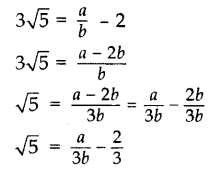Since a and b are integers, we get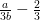is rational and so √5 is rational.
But this contradicts the fact that √5 is irrational.
So, we conclude that 2 + 3√5 is irrational.

Show that 3√7 is an irrational number. (2016)

જવાબ : Let us assume, to the contrary, that 3√7 is rational.
That is, we can find coprime a and b (b ≠ 0) such that 3√7 =Rearranging, we get √7 =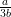Since 3, a and b are integers,is rational, and so √7 is rational.
But this contradicts the fact that √7 is irrational.
So, we conclude that 3√7 is irrational.

Explain why (17 × 5 × 11 × 3 × 2 + 2 × 11) is a composite number? (2015)

જવાબ : 17 × 5 × 11 × 3 × 2 + 2 × 11 …(i)
= 2 × 11 × (17 × 5 × 3 + 1)
= 2 × 11 × (255 + 1)
= 2 × 11 × 256
Number (i) is divisible by 2, 11 and 256, it has more than 2 prime factors.
Therefore (17 × 5 × 11 × 3 × 2 + 2 × 11) is a composite number.

Check whether 4n can end with the digit 0 for any natural number n. (2015)

જવાબ : 4n = (22)n = 22n
The only prime in the factorization of 4n is 2.
There is no other prime in the factorization of 4n = 22n
(By uniqueness of the Fundamental Theorem of Arithmetic).
5 does not occur in the prime factorization of 4n for any n.
Therefore, 4n does not end with the digit zero for any natural number n.

Can two numbers have 15 as their HCF and 175 as their LCM? Give reasons. (2017 OD)

જવાબ : No, LCM = Product of the highest power of each factor involved in the numbers.
HCF = Product of the smallest power of each common factor.
We can conclude that LCM is always a multiple of HCF, i.e., LCM = k × HCF
We are given that,
LCM = 175 and HCF = 15
175 = k × 15
⇒ 11.67 = k
But in this case, LCM ≠ k × HCF
Therefore, two numbers cannot have LCM as 175 and HCF as 15.

Identify whether Rational or Irrational it is : √15

જવાબ : Irrational No.

Identify whether Rational or Irrational it is : √-1

જવાબ : Neither Rational nor Irrational no.

Identify whether Rational or Irrational it is : 1.365835267495……

જવાબ : Irrational No.

Identify whether Rational or Irrational it is :  (√7)2

જવાબ : Rational No.

Identify whether Rational or Irrational it is : √199

જવાબ : Irrational No.

Identify whether Rational or Irrational it is : √121

જવાબ : Rational No.

Identify whether Rational or Irrational it is : √18

જવાબ : Irrational No.

Identify whether Rational or Irrational it is :  √16

જવાબ : RationalNo.

Answer the following in one word :- Numbers of the forms p/q

જવાબ : Rational

Answer the following in one word :- In Euclid division lemma a=bq + r , it is the value b

જવાબ : quotient

Answer the following in one word :- HCF can be found using this division algorithm

જવાબ : Euclid

Answer the following in one word :- In Euclid division lemma a=bq + r , it is the value r

જવાબ : remainder

Answer the following in one word :- Number which can be written as product of prime

જવાબ : Composite

Answer the following in one word :- Number which are not divisible by any other number except 1

જવાબ : Prime No.

Answer the following in one word :-  decimal expression can be expressed in the form 1/2m5n

જવાબ : Terminating

Find the nature of the product (√2−√3)(√3+√2)(2−3)(3+2) ?

જવાબ : (√2−√3)(√3+√2)(2−3)(3+2)
=(√2−√3)(√2+√3)=(2−3)(2+3)
=2-3=-1
So, it is rational number

Find the HCF and LCM by factorization technique 29029 ,580

જવાબ : 29029=29×13×11×729029=29×13×11×7
580=29×5×4580=29×5×4
HCF=29
LCM=29×13×11×7×4×5=58058029×13×11×7×4×5=580580

Find the HCF and LCM by factorization technique 120 ,144

જવાબ : 120=2×2×3×2×5120=2×2×3×2×5
144=2×2×3×2×2×3144=2×2×3×2×2×3
HCF=23×3=24HCF=23×3=24
LCM=720

Find the HCF and LCM by factorization technique 27,81

જવાબ : 27=3×3×327=3×3×3
81=3×3×3×381=3×3×3×3
HCF=27
LCM=81

A rational number can be expressed as terminating decimal when the factors of the denominator are _______

જવાબ : 2 & 5

if a and b are co primes, LCM (a, b) = ______

જવાબ : axb

the HCF (a, b) =2 and LCM (a, b) =27. What is the value a×ba×b

જવાબ : 54

State whether the statement is true or false :- Number of the form 2n+12n+1 where n is any positive integer are always odd number

જવાબ : True

State whether the statement is true or false :- Product of two prime number is always equal to their LCM

જવાબ : True

State whether the statement is true or false :- Any positive odd integer is of the form 6m+1 or 6m+3 or 6m +5 where q is some integer

જવાબ : True

State whether the statement is true or false :-  The number 6n for n in natural number can end in digit zero

જવાબ : False

State whether the statement is true or false :- √3 Can be expressed in the form √3/1,so it is a rational number

જવાબ : False

State whether the statement is true or false :- There are finite rational number between 2 and 3

જવાબ : False

State whether the statement is true or false :- There are infinite integers between two integers

જવાબ : False

State whether the statement is true or false :- The HCF of two prime number is always 1

જવાબ : True

State whether the statement is true or false :- Every integer is a rational number

જવાબ : True

State whether the statement is true or false :- √3×√123×12 is a irrational number

જવાબ : False

Prove that √5 is irrational and hence show that 3 + √5 is also irrational. (2012)

જવાબ : Let us assume, to the contrary, that √5 is rational.
So, we can find integers p and q (q ≠ 0), such that
√5 =, where p and q are coprime.
Squaring both sides, we get
5 =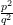5q2 = p2 …(i)
5 divides p2
5 divides p
So, let p = 5r
Putting the value of p in (i), we get
5q2 = (5r)2
5q= 25r2
q2 = 5r2
5 divides q2
5 divides q
So, p and q have atleast 5 as a common factor.
But this contradicts the fact that p and q have no common factor.
So, our assumption is wrong, is irrational.
√5 is irrational, 3 is a rational number.
So, we conclude that 3 + √5 is irrational.

Prove that 3 + 2√3 is an irrational number. (2014)

જવાબ : Let us assume to the contrary, that 3 + 2√3 is rational.
So that we can find integers a and b (b ≠ 0).
Such that 3 + 2√3 =, where a and b are coprime.
Rearranging the equations, we get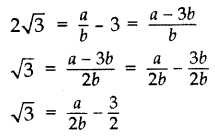Since a and b are integers, we get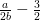is rational and so √3 is rational.
But this contradicts the fact that √3 is irrational.
So we conclude that 3 + 2√3 is irrational.

Three bells toll at intervals of 9, 12, 15 minutes respectively. If they start tolling together, after what time will they next toll together? (2013)

જવાબ : 9 = 32, 12 = 22 × 3, 15 = 3 × 5
LCM = 22 × 32 × 5 = 4 × 9 × 5 = 180 minutes or 3 hours
They will next toll together after 3 hours.

Two tankers contain 850 liters and 680 liters of petrol. Find the maximum capacity of a container which can measure the petrol of each tanker in the exact number of times. (2012)

જવાબ : To find the maximum capacity of a container which can measure the petrol of each tanker in the exact number of times, we find the HCF of 850 and 680.
850 = 2 × 52 × 17
680 = 23 × 5 × 17
HCF = 2 × 5 × 17 = 170
Maximum capacity of the container = 170 liters.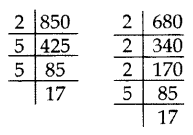​​​​​​​

The length, breadth, and height of a room are 8 m 50 cm, 6 m 25 cm and 4 m 75 cm respectively. Find the length of the longest rod that can measure the dimensions of the room exactly. (2015)

જવાબ : To find the length of the longest rod that can measure the dimensions of the room exactly, we have to find HCF.
L, Length = 8 m 50 cm = 850 cm = 21 × 52 × 17
B, Breadth = 6 m 25 cm = 625 cm = 54
H, Height = 4 m 75 cm = 475 cm = 52 × 19
HCF of L, B and H is 52 = 25 cm
Length of the longest rod = 25 cm

Three alarm clocks ring at intervals of 4, 12 and 20 minutes respectively. If they start ringing together, after how much time will they next ring together? (2015)

જવાબ : To find the time when the clocks will next ring together,
we have to find LCM of 4, 12 and 20 minutes.
4 = 22
12 = 22 × 3
20 = 22 × 5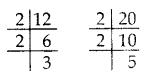LCM of 4, 12 and 20 = 22 × 3 × 5 = 60 minutes.
So, the clocks will ring together again after 60 minutes or one hour.

In a school, there are two Sections A and B of class X. There are 48 students in Section A and 60 students in Section B. Determine the least number of books required for the library of the school so that the books can be distributed equally among all students of each Section. (2017 OD)

જવાબ : Since the books are to be distributed equally among the students of Section A and Section B. therefore, the number of books must be a multiple of 48 as well as 60.
Hence, required num¬ber of books is the LCM of 48 and 60.
48 = 24 × 3
60 = 22 × 3 × 5
LCM = 24 × 3 × 5 = 16 × 15 = 240
Hence, required number of books is 240.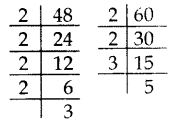​​​​​​​

Using Euclid’s division algorithm, find whether the pair of numbers 847, 2160 are coprime or not.
To find out the minimum (least) time when the bells toll together next, we find the LCM of 9, 12, 15.

જવાબ :By using Euclid’s algorithm, find the largest number which divides 650 and 1170. (2017 OD)

જવાબ : Given numbers are 650 and 1170.
1170 > 650
1170 = 650 × 1 + 520
650 = 520 × 1 + 130
520 = 130 × 4 + 0
HCF = 130
The required largest number is 130.

Find the HCF of 255 and 867 by Euclid’s division algorithm. (2014)

જવાબ : 867 is greater than 255. We apply the division lemma to 867 and 255, to get
867 = 255 × 3 + 102
We continue the process till the remainder is zero
255 = 102 × 2 + 51
102 = 51 × 2 + 0, the remainder is zero.
HCF = 51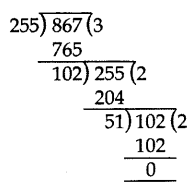Prove that 3 + 2√5 is irrational. (2012, 2017 D)

જવાબ : Let us assume, to the contrary, that 3 + 2√5 is rational
So that we can find integers a and b (b ≠ 0), such that
3 + 2 √5 =, where a and b are coprime.
Rearranging this equation, we get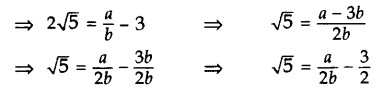Since a and b are integers, we get that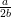–is rational and so √5 is rational.
But this contradicts the fact that √5 is irrational.
So we conclude that 3 + 2√5 is irrational.

There are 104 students in class X and 96 students in class IX in a school. In a house examination, the students are to be evenly seated in parallel rows such that no two adjacent rows are of the same class. (2013)

જવાબ : (a) Find the maximum number of parallel rows of each class for the seating arrange¬ment.
(b) Also, find the number of students of class IX and also of class X in a row.
(c) What is the objective of the school administration behind such an arrangement?
Solution:
104 = 23 × 13
96 = 25 × 3
HCF = 23 = 8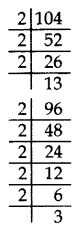(a) Number of rows of students of class X =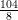= 13
Number maximum of rows class IX =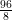= 12
Total number of rows = 13 + 12 = 25
(b) No. of students of class IX in a row = 8
No. of students of class X in a row = 8
(c) The objective of school administration behind such an arrangement is fair and clean examination, so that no student can take help from any other student of his/her class.

Dudhnath has two vessels containing 720 ml and 405 ml of milk respectively. Milk from these containers is poured into glasses of equal capacity to their brim. Find the minimum number of glasses that can be filled. (2014)

જવાબ : 1st vessel = 720 ml; 2nd vessel = 405 ml
We find the HCF of 720 and 405 to find the maximum quantity of milk to be filled in one glass.
405 = 34 × 5
720 = 24 × 32 × 5
HCF = 32 × 5 = 45 ml = Capacity of glass
No. of glasses filled from 1st vessel =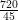= 16
No. of glasses filled from 2nd vessel =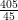= 9
Total number of glasses = 25

Amita, Sneha, and Raghav start preparing cards for all persons of an old age home. In order to complete one card, they take 10, 16 and 20 minutes respectively. If all of them started together, after what time will they start preparing a new card together? (2013)

જવાબ : To find the earliest (least) time, they will start preparing a new card together, we find the LCM of 10, 16 and 20.
10 = 2 × 5
16 = 24
20 = 22 × 5
LCM = 24 × 5 = 16 × 5 = 80 minutes
They will start preparing a new card together after 80 minutes.

Find HCF of numbers 134791, 6341 and 6339 by Euclid’s division algorithm. (2015)

જવાબ : First, we find HCF of 6339 and 6341 by Euclid’s division method.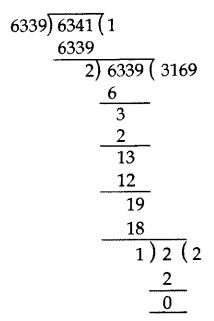6341 > 6339
6341 = 6339 × 1 + 2
6339 = 2 × 3169 + 1
2 = 1 × 2 + 0
HCF of 6341 and 6339 is 1.
Now, we find the HCF of 134791 and 1
134791 = 1 × 134791 + 0
HCF of 134791 and 1 is 1.
Hence, the HCF of the given three numbers is 1.

If two positive integers x and y are expressible in terms of primes as x = p2q3 and y = p3q, what can you say about their LCM and HCF. Is LCM a multiple of HCF? Explain. (2014)

જવાબ : x = p2q3 and y = p3q
LCM = p3q3
HCF = p2q …..(i)
Now, LCM = p3q3
LCM = pq2 (p2q)
LCM = pq2 (HCF)
Yes, LCM is a multiple of HCF.
Explanation:
Let a = 12 = 22 × 3
b = 18 = 2 × 32
HCF = 2 × 3 = 6 …(ii)
LCM = 22 × 32 = 36
LCM = 6 × 6
LCM = 6 (HCF) …[From (ii)]
Here LCM is 6 times HCF.

Show that one and only one out of n, (n + 1) and (n + 2) is divisible by 3, where n is any positive integer. (2015)

જવાબ : Let n, n + 1, n + 2 be three consecutive positive integers.
We know that n is of the form 3q, 3q + 1, or 3q + 2.
Case I. When n = 3q,
In this case, n is divisible by 3,
but n + 1 and n + 2 are not divisible by 3.
Case II. When n = 3q + 1,
In this case n + 2 = (3q + 1) + 2
= 3q + 3
= 3(q + 1 ), (n + 2) is divisible by 3,
but n and n + 1 are not divisible by 3.
Case III.
When n = 3q + 2, in this case,
n + 1 = (3q + 2) + 1
= 3q + 3 = 3 (q + 1 ), (n + 1) is divisible by 3,
but n and n + 2 are not divisible by 3.
Hence, one and only one out of n, n + 1 and n + 2 is divisible by 3.

Find the HCF and LCM of 306 and 657 and verify that LCM × HCF = Product of the two numbers. (2016 D)

જવાબ : 306 = 2 × 32 × 17
657 = 32 × 73
HCF = 32 = 9
LCM = 2 × 32 × 17 × 73 = 22338
L.H.S. = LCM × HCF = 22338 × 9 = 201042
R.H.S. = Product of two numbers = 306 × 657 = 201042
L.H.S. = R.H.S.

Show that any positive odd integer is of the form 41 + 1 or 4q + 3 where q is a positive integer. (2016 OD)

જવાબ : Let a be a positive odd integer
By Euclid’s Division algorithm:
a = 4q + r …[where q, r are positive integers and 0 ≤ r < 4]
a = 4q
or 4q + 1
or 4q + 2
or 4q + 3
But 4q and 4q + 2 are both even
a is of the form 4q + 1 or 4q + 3.

Use Euclid’s division lemma to show that the square of any positive integer is either of the form 3n or 3n + 1 for some integer m.

જવાબ : Let x be any positive integer and y = 3.

By Euclid’s division algorithm;

x =3q + r (for some integer q ≥ 0 and r = 0, 1, 2 as r ≥ 0 and r < 3)

Therefore,

x = 3q, 3q + 1 and 3q + 2

As per the given question, if we take the square on both the sides, we get;

x2 = (3q)2 = 9q2 = 3.3q2

Let 3q2 = n

Therefore,

x2 = 3n ………………….(1)

x2 = (3q + 1)2

= (3q)2 + 12 + 2 × 3q × 1

= 9q2 + 1 + 6q

= 3(3q2 + 2q) + 1

Substitute, 3q2+2q = n, to get,

x2 = 3n + 1 ……………………………. (2)

x2 = (3q + 2)2

= (3q)+ 2+ 2 × 3q × 2

= 9q2 + 4 + 12q

= 3(3q2 + 4q + 1) + 1

Again, substitute, 3q+ 4q + 1 = m, to get,

x2 = 3n + 1…………………………… (3)

Hence, from eq. 1, 2 and 3, we conclude that, the square of any positive integer is either of the form 3n or 3n + 1 for some integer n.

Express each number as a product of its prime factors:
(i) 1400
(ii) 1560
(iii) 38250
(iv) 50050
(v) 74290

જવાબ : (i) 1400
140 = 2 × 2 × 2 × 5 x 5 × 7 × 1

(ii) 1560

156 = 2 × 2 × 2 × 5 x 13 × 3

(iii) 38250

3825 = 2  x 3 × 3 × 5 × 5 × 5 × 17

(iv) 50050

5005 = 2 × 5 x 5 × 7 × 11 × 13

(v) 74290

7429 = 2 × 5 x 17 × 19 × 23

### There are No Content Availble For this Chapter

 1 Rational No A Root of -1 2 Irrational No. B Rational & Irrational no. 3 Real No. C 1/3 4 Non-Real No. D Square root of 2

જવાબ :

1-C , 2-D, 3-B, 4-A

 1 Whole No. A 1/7 2 Natural No B 0,1,2,3…… 3 Integers. C 1,2,3,4…… 4 Fractions D …-2,-1,0,1,2…

જવાબ :

1-B, 2-C, 3-D, 4-A

 1 Even No. A 4,6,8,9,12 2 Odd No . B 2,3,5,7,11 3 Prime No. C 1,3,5,7,11 4 Composite No. D 2,4,6,8,10

જવાબ :

1-D, 2-C, 3-B, 4-A

 No. H.C.F. 1 6, 12, 15 A 1 2 4, 8, 16 B 3 3 10, 25, 50 C 4 4 2, 3, 4 D 5

જવાબ :

1-B,.  2-C, 3-D, 4-A

 No. L.C.M 1 12, 13 A 150 2 100, 15 B 156 3 50, 60 C 66 4 2, 33 D 300

જવાબ :

1-B, 2-A, 3-D, 4-C

 1 Rational No A √2 2 Irrational No. B √2 & √4 3 Real No. C √-4 4 Non-Real No. D √4

જવાબ :

1-D, 2-A, 3-B, 4-C

 1 Even No. A 32,34,35,36 2 Odd No . B 31,37,41,43 3 Prime No. C 32,34,36 4 Composite No. D 31,33,35,37

જવાબ :

1-C, 2-D, 3-B, 4-A

 No. H.C.F. 1 33,100 A 4 2 44, 46 B 3 3 3, 39 C 2 4 8,12 D 1

જવાબ :

1-D, 2-C, 3-B, 4-A

 No. L.C.M. 1 33,100 A 24 2 44, 46 B 3300 3 3, 39 C 903 4 8,12 D 39

જવાબ :

1-B, 2-C, 3-D, 4-A

 No. H.C.F. 1 12, 13 A 5 2 100, 15 B 2 3 50, 60 C 1 4 2, 32 D 10

જવાબ :

1-C,2-A,3-D,4-B